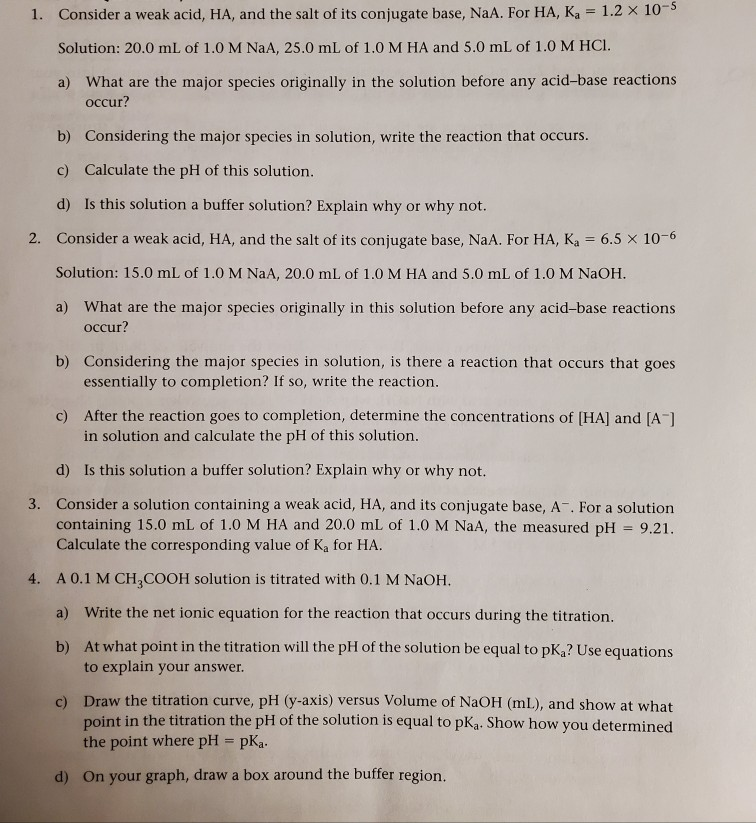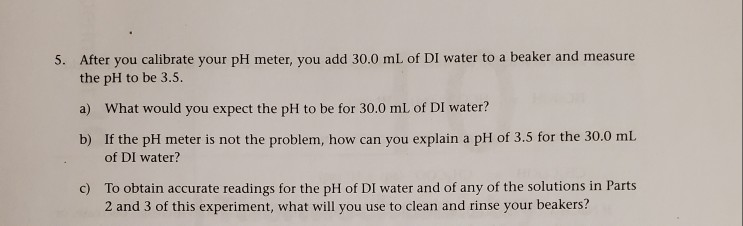To add more information this was given to me for a lab that used a weak acid and we added a strong base through titration. We just observed how the ph changes. Later we then used a buffer with a weak acid to see how buffers affect ph change. These questions are basically surrounded around those topics to help us prepare. However, I'm kinda confused about answering them because weak acid and their conjugate bases confuse me. So please can you help me out?

1. Consider a weak acid, HA, and the salt of its conjugate base, NaA. For HA, K = 1.2 x 100 Solution: 20.0 mL of 1.0 M NaA, 25.0 mL of 1.0 MHA and 5.0 mL of 1.0 M HCI. a) What are the major species originally in the solution before any acid-base reactions occur? b) Considering the major species in solution, write the reaction that occurs. c) Calculate the pH of this solution. d) Is this solution a buffer solution? Explain why or why not. 2. Consider a weak acid, HA, and the salt of its conjugate base, NaA. For HA, K = 6.5 x 10-6 Solution: 15.0 mL of 1.0 M NaA, 20.0 mL of 1.0 MHA and 5.0 mL of 1.0 M NaOH. a) What are the major species originally in this solution before any acid-base reactions occur? b) Considering the major species in solution, is there a reaction that occurs that goes essentially to completion? If so, write the reaction. c) After the reaction goes to completion, determine the concentrations of (HA) and [A] in solution and calculate the pH of this solution. d) Is this solution a buffer solution? Explain why or why not. 3. Consider a solution containing a weak acid, HA, and its conjugate base, A. For a solution containing 15.0 mL of 1.0 M HA and 20.0 mL of 1.0 M NaA, the measured pH = 9.21. Calculate the corresponding value of K for HA. A 0.1 M CH,COOH solution is titrated with 0.1 M NaOH. a) Write the net ionic equation for the reaction that occurs during the titration. b) At what point in the titration will the pH of the solution be equal to pK? Use equations to explain your answer. c) Draw the titration curve, pH (y-axis) versus Volume of NaOH (ml), and show at what point in the titration the pH of the solution is equal to pKg. Show how you determined the point where pH = pka. d) On your graph, draw a box around the buffer region.
5. After you calibrate your pH meter, you add 30.0 mL of DI water to a beaker and measure the pH to be 3.5. a) What would you expect the pH to be for 30.0 mL of DI water? b) If the pH meter is not the problem, how can you explain a pH of 3.5 for the 30.0 mL of DI water? c) To obtain accurate readings for the pH of DI water and of any of the solutions in Parts 2 and 3 of this experiment, what will you use to clean and rinse your beakers?

In the fifth question, the meter is showing pH of 3.50 when distilled water was taken in the beaker because, may be the beaker was not washed properly some sort of acid either hcl or ch3cooh had been there in the beaker.

Distilled water has a oh of 0 as it does not contains negligible amount of H+ .

To obtain accurate readings of distilled water and that of solutions in part second and third the beaker has to be rinsed properly with that solution which has to taken in the beaker otherwise flawed observation occurs.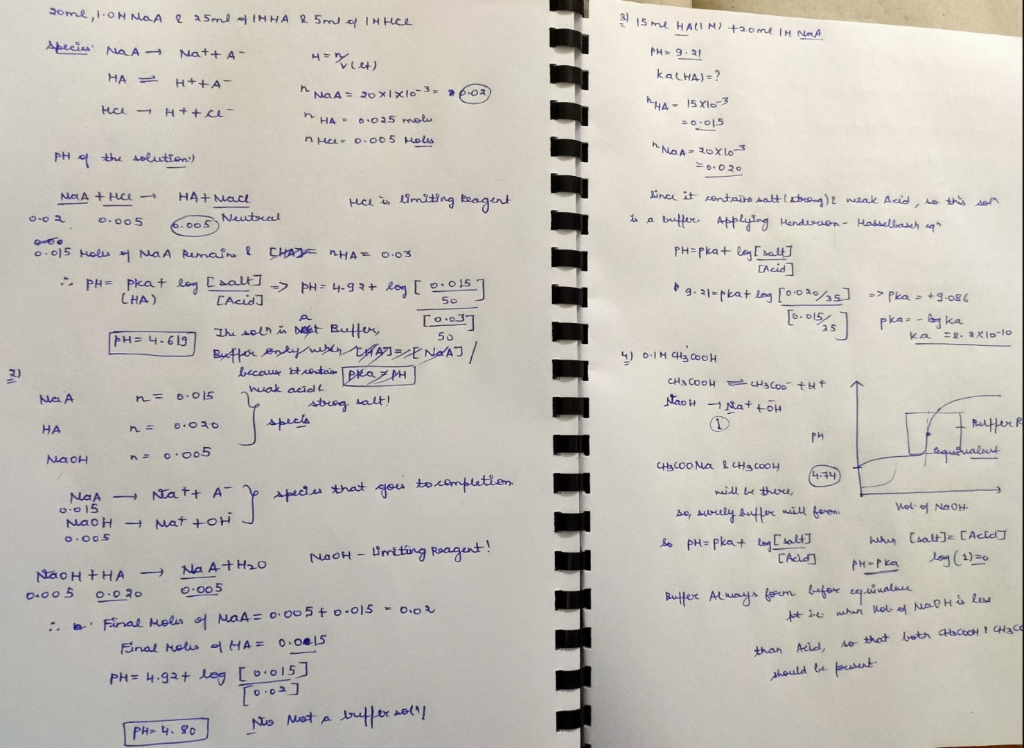#### Earn Coin

Coins can be redeemed for fabulous gifts.

Similar Homework Help Questions
• ### please help me with these questions. Thank you! Classify each titration curve as representing a strong...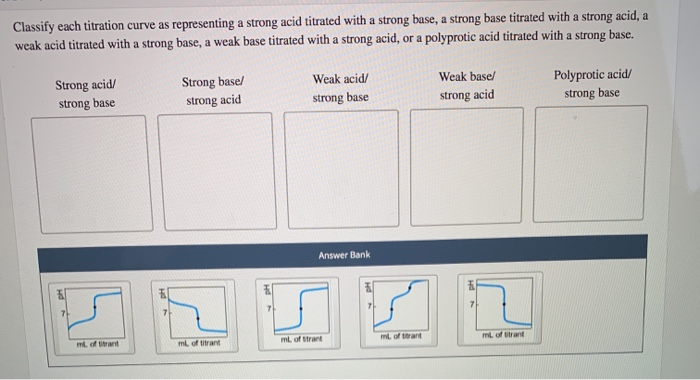please help me with these questions. Thank you! Classify each titration curve as representing a strong acid titrated with a strong base, a strong base titrated with a strong acid, a weak acid titrated with a strong base, a weak base titrated with a strong acid, or a polyprotic acid titrated with a strong base. Strong acid/ strong base Strong base/ strong acid Weak acid/ strong base Weak based strong acid Polyprotic acid strong base Answer Bank Calculate the pH...can someone please help me figure out these 5 questions with the calculations shown please. and i need help with the graph ?? Acid-Base Titration of a Weak Acid with a Strong Base: Determination of K. Introduction: You will be titrating a solution of a weak acid with 0.100 M NaOH, while monitoring the reaction using a pH meter. Weak acids have characteristic acid-ionization constants, K. The purpose of this lab is to use the titration to determine the value...

• ### can someone help me answer these 5 questions and figire this graph out please? Acid-Base Titration...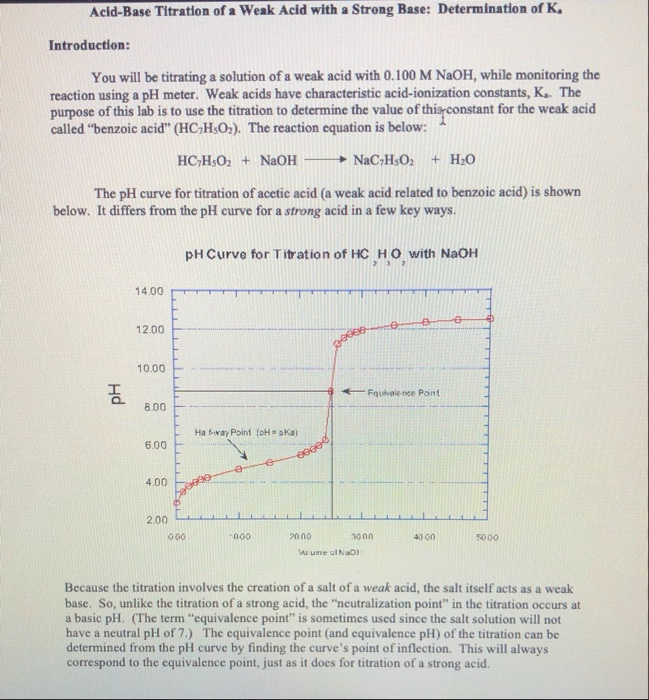can someone help me answer these 5 questions and figire this graph out please? Acid-Base Titration of a Weak Acid with a Strong Base: Determination of K. Introduction: You will be titrating a solution of a weak acid with 0.100 M NaOH, while monitoring the reaction using a pH meter. Weak acids have characteristic acid-ionization constants, K. The purpose of this lab is to use the titration to determine the value of this constant for the weak acid called “benzoic...

• ### A student peforms a titration, titrating 25.00 mL of a weak monoprotic acid, HA, with a...

A student peforms a titration, titrating 25.00 mL of a weak monoprotic acid, HA, with a 1.22 M solution of NaOH. They collect data, plot a titration curve and determine the values given in the below table. ml NaOH added pH Half-way Point 18.34 4.06 Equivalence point 36.68 8.84 How many moles of NaOH have been added at the equivalence point? mol What is the total volume of the solution at the equivalence point? mL During the titration the following...

• ### 17. The hydrogen sulfate or bisulfate ion HSO4 can act as either an acid or a...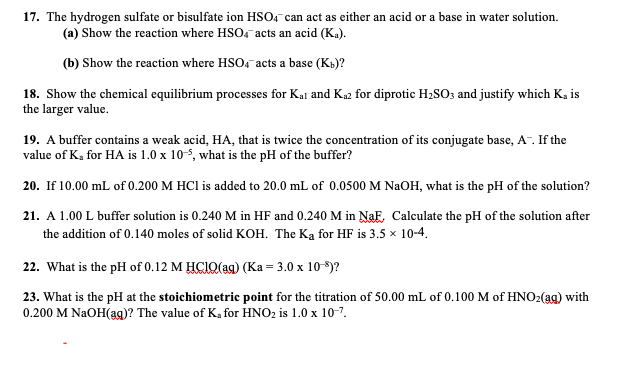17. The hydrogen sulfate or bisulfate ion HSO4 can act as either an acid or a base in water solution (a) Show the reaction where HSO4 acts an acid (Ka) (b) Show the reaction where HSO4 acts a base (Kb)? 18. Show the chemical equilibrium processes for Kal and Ka2 for diprotic H2SO3 and justify which Ka is the larger value 19. A buffer contains a weak acid, HA, that is twice the concentration of its conjugate base, A. If...

• ### A student peforms a titration, titrating 25.00 mL of a weak monoprotic acid, HA, with a...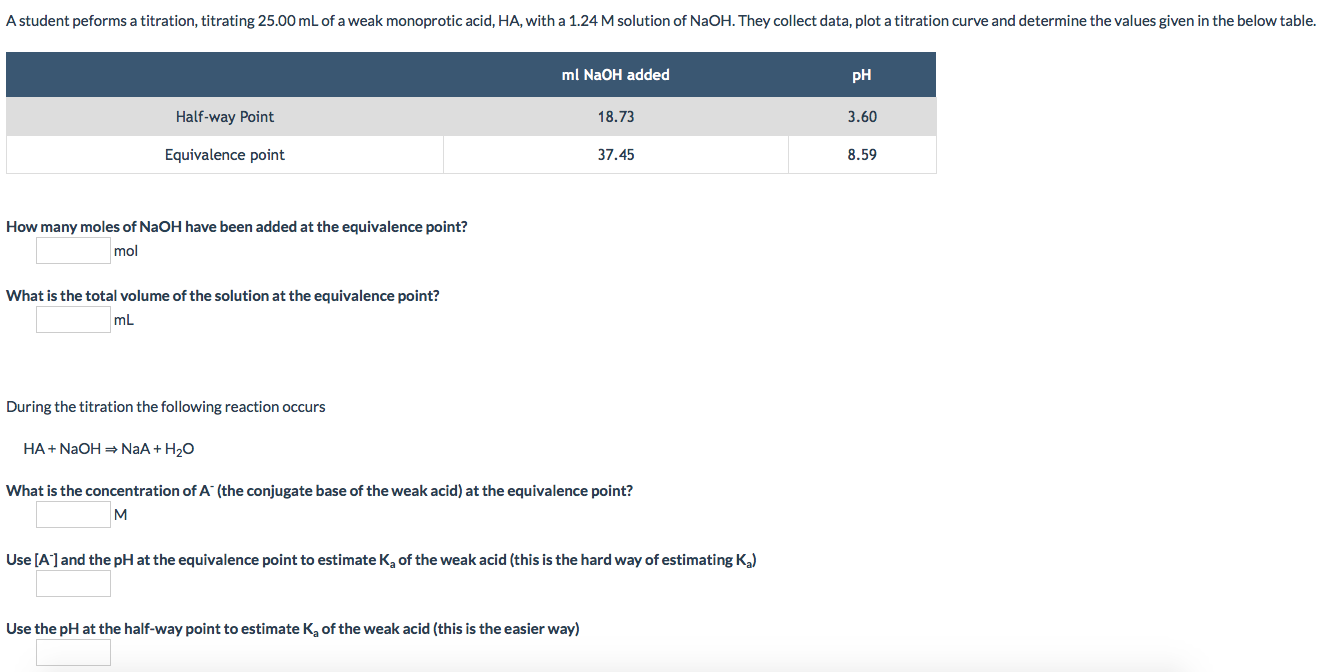A student peforms a titration, titrating 25.00 mL of a weak monoprotic acid, HA, with a 1.24 M solution of NaOH. They collect data, plot a titration curve and determine the values given in the below table. ml NaOH added pH Half-way Point 18.73 3.60 Equivalence point 37.45 8.59 How many moles of NaOH have been added at the equivalence point? mol What is the total volume of the solution at the equivalence point? mL During the titration the following...

• ### A student peforms a titration, titrating 25.00 mL of a weak monoprotic acid, HA, with a...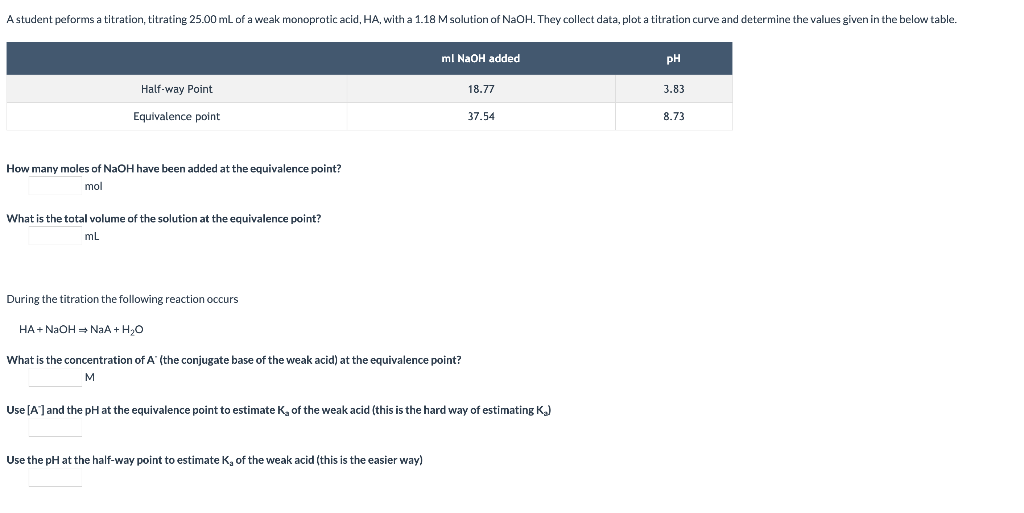A student peforms a titration, titrating 25.00 mL of a weak monoprotic acid, HA, with a 1.18 M solution of NaOH. They collect data, plot a titration curve and determine the values given in the below table. ml NaOH added pH Half-way Point 18.77 3.83 Equivalence point 37.54 8.73 How many moles of NaOH have been added at the equivalence point? mol What is the total volume of the solution at the equivalence point? mL During the titration the following...

• ### During the titration the following reaction occurs HA + NaOH = NaA+H2O What is the concentration...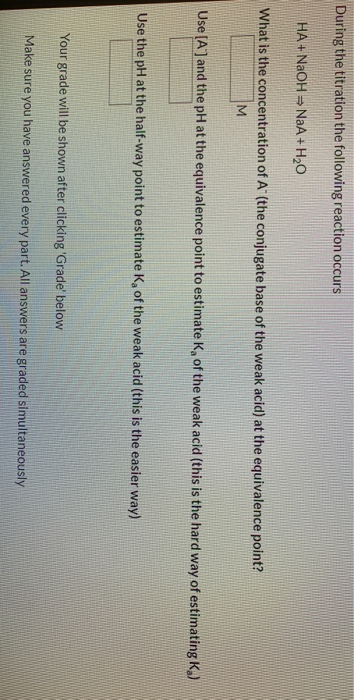During the titration the following reaction occurs HA + NaOH = NaA+H2O What is the concentration of A (the conjugate base of the weak acid) at the equivalence point? M Use [A] and the pH at the equivalence point to estimate Ko of the weak acid (this is the hard way of estimating K.) Use the pH at the half-way point to estimate of the weak acid (this is the easier way) Your grade will be shown after clicking 'Grade'...

• ### Titration of weak acid with strong base pre-lab Answers can only be entered/graded between 2019-10-24 and...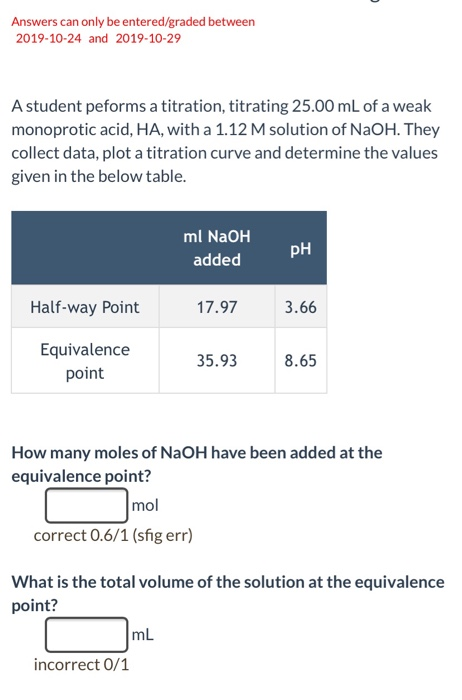Titration of weak acid with strong base pre-lab Answers can only be entered/graded between 2019-10-24 and 2019-10-29 A student peforms a titration, titrating 25.00 mL of a weak monoprotic acid, HA, with a 1.12 M solution of NaOH. They collect data, plot a titration curve and determine the values given in the below table. ml NaOH PH added Half-way Point 17.97 3.66 Equivalence point 35.938.65 How many moles of NaOH have been added at the equivalence point? r mol correct...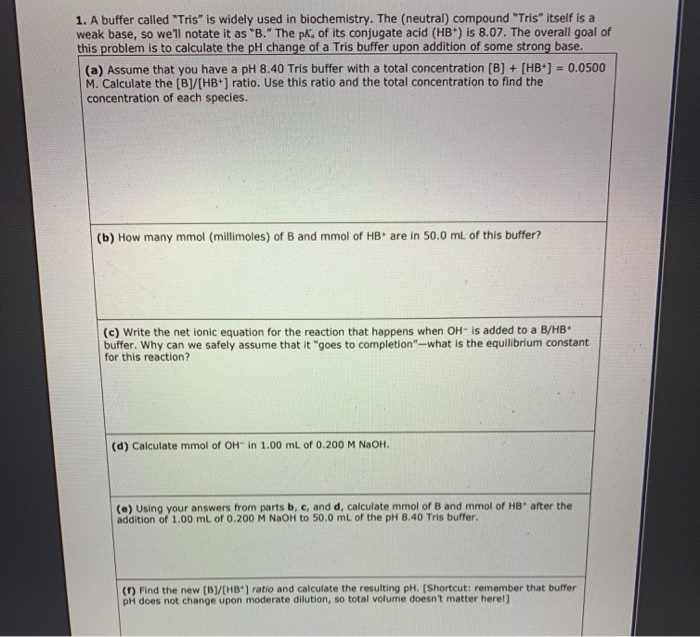Can someone please help me with this? I already asked this and nobody answered. 1. A buffer called "Tris" is widely used in biochemistry. The (neutral) compound "Tris" itself is a weak base, so we'll notate it as "B." The pk, of its conjugate acid (HB) is 8.07. The overall goal of this problem is to calculate the pH change of a Tris buffer upon addition of some strong base. (a) Assume that you have a pH 8.40 Tris buffer...